# KCET 2019 Chemistry Paper with Solutions

KCET is conducted by Karnataka Examination Authority (KEA) for admission to various engineering, pharmacy, agriculture courses and veterinary courses, in different colleges in the state of Karnataka. Students are recommended to practice KCET 2019 Chemistry paper solutions so that they can improve their speed of solving problems. BYJU’S provides detailed solutions that are prepared by subject experts. These solutions will help candidates to get familiar with the question paper and the question pattern, understand the marking scheme and more. Students can easily download the solutions in PDF format from below.

### KCET 2019 - Chemistry

Question 1: Which of the following possess net dipole moment?

1. a. BF3
2. b. SO2
3. c. CO2
4. d. BeCl2

Solution:

By seeing geometry, we can easily find out the net dipole moment BeCl2 and CO2 have linear geometry, BF3 has triangular planar geometry with zero dipole moment. While SO2 geometry is in a bent shape.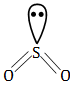Question 2: The number of -bonds and -bonds present in naphthalene are respectively

1. a. 5, 19
2. b. 6, 19
3. c. 5, 20
4. d. 5, 11

Solution:

We can find out by drawing the structure of Naphthalene.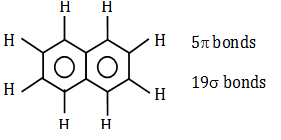The number of -bonds and -bonds are 5, 19 respectively.

Question 3: The reaction in which is H>U is

1. a. CaCO3(s) CaO(s) + CO2(g)
2. b. N2(g) +O2(g) 2NO(g)
3. c. CH4(g)+2O2(g) CO2(g) +2H2O(l)
4. d. N 2(g) + 3H2(g) 2NH3(g)

Solution:

In the reaction

CaCO3(s) CaO(S) + CO2(g)

Here n = +1

H = U + ngRT

So, H > U

Question 4: The number of moles of electron required to reduce 0.2 mole of Cr2O7-2 to Cr+3

1. a. 6
2. b. 1.2
3. c. 0.6
4. d. 12

Solution:

Cr2+6O72- 2Cr+3

Here one mole Cr2O72– requires 6 mole of electrons 0.2 mol requires (0.2×6)/1 = 1.2 mole of electrons.

Question 5: In reaction B(OH)3 + 2H2O ->[B(OH)4] + H3O+ ; B(OH)3 functions as

1. a. Lewis base
2. b. Protonic acid
3. c. Lewis acid
4. d. Bronsted acid

Solution:

Boric acid is a weak acid it acts as Lewis acid by accepting electrons from OH- ions.

Question 6: Match the following acids with their pKa values: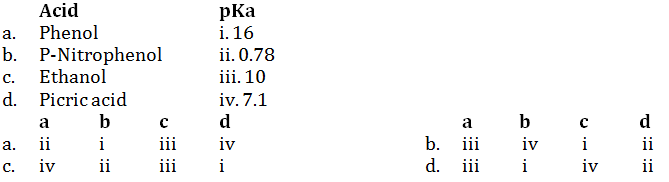Solution:

The compound will be more acidic when its conjugate base is more stable. In case of these given compounds, more the electron with the drawing group, present more the compound will be more acidic.

Question 7: Which of the following can be used to test the acidic nature of ethanol?

1. a. Na2CO3
2. b. Blue litmus solution
3. c. Na metal
4. d. NaHCO3

Solution:

Sodium metal can be used because when sodium metal reacts with ethanol, H2 gas is evolved.

C2H5OH +Na C2H5ONa+(1/2)H2

Question 8: The reagents A, B and C respectively are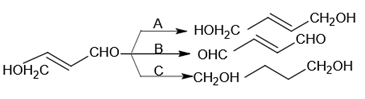1. a. NaBH4, alk. KMnO4, H2/Pd
2. b. H2/Pd, PCC, NaBH4
3. c. H2/Pd, alk. KMnO4, NaBH4
4. d. NaBH4, PCC, H2/Pd

Solution:

Reagent A should be such that it reduces aldehyde to alcohol but do not affect the double bond. Reagent B should be such that it oxidizes alcohol to aldehyde without affecting double bond. Reagent C should be such that it reduces aldehyde to alcohol and double bond to sigma bond.

PCC and NaBH4 do not affect the double bond.

Question 9: Propanoic acid undergoes HVZ reaction to give chloropropanoic acid. The product obtained is

1. a. As stronger as propanoic acid
2. b. Stronger acid than propanoic acid
3. c. Stronger than dichloropropanoic acid
4. d. Weaker acid than propanoic acid

Solution:

Since in question the product is already mentioned. The product formed is chloropropanoic acid. Therefore a chlorine atom is also attached to a molecule which acts as EWG and increases acidic strength.

Question 10: R and S form benzyl benzoate when treated with each other. Hence P is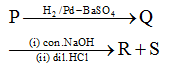1. a. C6H5CH2OH
2. b. C6H5CHO
3. c. C6H5COOH
4. d. C6H5COCl

Solution: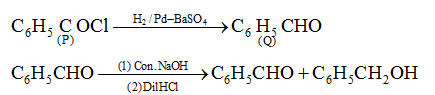Question 11: Among the following, the main reactions occurring in a blast furnace during the extraction of iron from haematite are;

i. Fe2O3 + 3CO 2Fe + 3CO2

ii. FeO + SiO2 FeSiO3

iii. Fe2O3 + 3C 2Fe + 3CO

iv. CaO + SiO2 CaSiO3

1. a. iii and iv
2. b. i and ii
3. c. i and iv
4. d. ii and iii

Solution:

The reactions that occur in a blast furnace during the extraction of iron are:

Fe2O3+3CO 2Fe+3CO2

CaO+SiO2 CaSiO3

Question 12: Which of the following pair contains 2 lone pair of electrons on the central atom?

1. a. H2O, NF3
2. b. I3+, H2O
3. c. SO4 2–, H2S
4. d. XeF4, NH3

Solution:

The structures of I3+ and H2O are: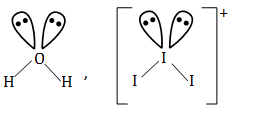They contain 2 lone pair of electrons on the central atom.

Question 13: Which of the following statement is correct?

1. a. Cl2 is a stronger oxidizing agent than F2
2. b. Cl2 oxidises H2O to O2 but F2 does not
3. c. Fluoride is a good oxidizing agent
4. d. F2 oxidises H2O to O2 but Cl2 does not

Solution:

2F2(g)+2H2O(l) 4H+ (aq)+4F(aq) +O2(g)

X2(g) +H2O(l) HX(aq) + HOX (aq)

x = Cl2 or Br2

So, by seeing above reactions we can conclude that F2 oxidizes H2O to O2 but Cl2 does not.

Question 14: 0.1 mole of XeF6 is treated with 1.8g of water. The product obtained is

1. a. XeO2F2
2. b. XeO3
3. c. Xe+XeO3
4. d. XeOF4

Solution: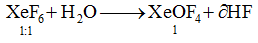0.1 Mole of XeF6 reacts with 0.1 mol of H2O (1.8 g) to give XeOF4.

Question 15: In the reaction of gold with aqua regia, the oxidation state of Nitrogen changes from

1. a. +6 to +4
2. b. +4 to +2
3. c. +3 to +1
4. d. +5 to +2

Solution:

Writing the equation of the reaction between gold and aqua regia;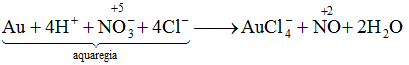Here, the oxidation state changes from +5 to +2.

Question 16: The vitamin that helps in clotting of blood is

1. a. C
2. b. A
3. c. K
4. d. B2

Solution:

Vitamin K helps in the clotting of blood. During injuries when blood flow occurs then vitamin k is responsible for clotting the blood and stopping it from flowing out from our body.

Question 17: The polymer containing five methylene groups as it’s repeating unit is;

1. a. Nylon 6
2. b. Nylon 6, 6
3. c. Bakelite
4. d. Dacron

Solution:

Nylon-6, the monomer has five methyl groups. H2N-CH2-CH2-CH2-CH2-CH2-COOH.

Question 18: Cis-1, 4-polyisoprene is called

1. a. Neoprene
2. b. Buna-N
3. c. Natural rubber
4. d. Buna-S

Solution:

Cis-1,4-polyisoprene, which is also called isoprene rubber, is a major ingredient of natural rubber.

Question 19: Which cleansing agent gets precipitated in hard water?

1. a. Sodium stearate
2. b. Sodium lauryl sulphate
3. c. Sodium dodecylbenzene sulphonate
4. d. Cetyl trimethyl ammonium bromide

Solution:

When soap reacts with hard water containing impurities of calcium and magnesium then scum of insoluble calcium stearate is formed.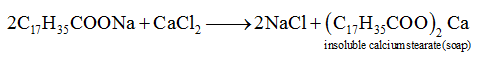Question 20:. Anti-histamine among the following is

1. a. Morphine
2. b. Bromopheneramine
3. c. Chloroxylenol
4. d. Amoxycillin

Solution:

Brompheniramine is an antihistamine. Antihistamines are drugs which treat allergic rhinitis and other allergies.

Question 21:. The elements in which electrons are progressively filled in 4f orbital are called

1. a. Transition elements
2. b. Actinoids
3. c. Halogens
4. d. Lanthanoids

Solution:

In lanthanoid the electrons are filled in 4f orbitals, while in halogens they are filled in p-orbitals and in transition elements, electrons are progressively filled in d-orbitals.

Question 22:. Incorrect statement with reference to Ce(Z = 58)

1. a. Ce in +3 oxidation state is more stable than in +4
2. b. Ce4+ is a reducing agent
3. c. Ce shows common oxidation states of +3 and +4
4. d. Atomic size of Ce is more than that of Lu

Solution:

Cl+4 is an oxidizing agent since it cannot attain oxidation state higher than +4 due to decrease of stability.

Question 23:. A mixture of NaCl and K2Cr2O7 is heated with conc. H2SO4, deep red vapours are formed. Which of the following statement is false?

1. a. The vapours contain CrO2Cl2 only
2. b. The vapours give a yellow solution with NaOH
3. c. The vapours when passed into lead acetate in acetic acid give a yellow precipitate
4. d. The vapours contain CrO2Cl2 and Cl2

Solution:

The given reaction is of chromyl chloride test

4Cl + Cr2O7 2–(s) + 6H+(conc.) 2CrO2Cl2 + 3H2O.

When deep red vapours of CrO2Cl2 are passed into a sodium hydroxide solution, a yellow solution of sodium chromate is formed, which when treated with lead acetate gives a yellow precipitate of lead chromate.

CrO2Cl2 + OH- CrO42– +2Cl +2H2O

CrO4 2– + Pb2+ PbCrO4 () yellow.

Question 24:. Which of the following statement is wrong?

1. a. Mn3+ and Co3+ are oxidizing agents in aqueous solution
2. b. In the highest oxidation states, the transition metals show acidic character
3. c. All element of 3d series exhibit variable oxidation states
4. d. Metals in highest oxidation states are more stable in oxides than in fluorides

Solution:

Zinc contains completely filled d-orbitals and exhibits stable electronic configuration, therefore, it does not easily lose their electrons and hence does not exhibit variable valency.

Question 25:. Which among the following is the strongest ligand?

1. a. NH3
2. b. CN
3. c. en
4. d. CO

Solution:

Here CO is the strongest ligand, due to the synergic effect of and bond. In this effect, some bonds are self-strengthening in nature, when a pi-back donation of electron density occurs from one atomic orbital of one atom to the anti-bonding pi-orbital of another.

Question 26:. Which of the following is a network crystalline solid?

1. a. AlN
2. b. I2
3. c. Ice
4. d. NaCl

Solution:

In a network solid or covalent network atoms are bonded by covalent bonds in a continuous network extending throughout the material. In network solid, there are no individual molecules and the entire crystal considered as macromolecules.

Question 27:. The number of atoms in 2.4 g of body centred cubic crystal with edge length 200 pm is (density = 10g cm–3, NA = 6×1023 atoms/mol)

1. a. 6 × 1020
2. b. 6 × 1022
3. c. 6 × 1019
4. d. 6 × 1023

Solution:

Using formula d = zm/a3NA

m = (d×a3×NA)/z = (10×2003×10-30×6×1023)/2

= 24

24g contains 6×1023

2.4 g will contain 6×1022

Question 28:. 1 mole of NaCl is doped with 10-5 mole of SrCl2. The number of cationic vacancies in the crystal lattice will be

1. a. 6.022 × 1015
2. b. 6.022 × 1018
3. c. 12.044 × 1020
4. d. 6.022 × 1023

Solution:

1 mole SrCl2 gives 1 cationic valancy 10-5 moles of SrCl2 gives 10-5 mole cationic valancy.

Therefore, the number of cationic vacancies in 1 mole of NaCl. When it is doped with 10-5 moles of SrCl2 is 6.022×1018.

Question 29:. A non-volatile solute, ‘A’ tetramerises in water to the extent of 80%, 2.5g of ‘A’ in 100g of water, lower the freezing point by 0.30C. The molar mass of A in mol L–1 is (Kf for water = 1.86 K kg mol–1)

1. a. 221
2. b. 62
3. c. 354
4. d. 155

Solution:

For applying formula of ΔTf we have to final α,

α= 80/100 = 0.8

α= (1-i)/(1-1/n)

α= (1-i)/(1-1/4)

α= (1-i)/(3/4)

i = 0.4

ΔTf = 0.3

0.3 = (i×Kf×w2×1000)/(m2×100)

m2 = 62g/mol

Question 30:. Solution ‘A’ contains acetone dissolved in chloroform and solution ‘B’ contains acetone dissolved in carbon disulphide. The type of deviations from Raoult’s law shown by solutions A and B, respectively are:

1. a. Positive and negative
2. b. Positive and positive
3. c. Negative and positive
4. d. Negative and negative

Solution:

Acetone-carbon disulphide solution shows a positive deviation from Raoult’s law. The observed vapour pressure of each component and total, vapour pressure is greater than predicted by Raoult’s law. Acetone + Chloroform give negative deviation.

Question 31:. The mass of AgCl precipitated when a solution containing 11.70g of NaCl is added to a solution containing 3.4g of AgNO3 is [Atomic mass of Ag = 108, Atomic mass of Na = 23]

1. a. 1.17 g
2. b. 5.74 g
3. c. 6.8
4. d. 2.87 g

Solution: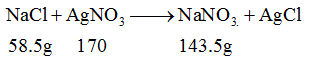(limiting reagent)

Required ratio 58.5: 170

Given ratio 11.70: 3.4 (Limiting reagent)

170g AgNO3 143.5 (g) AgCl

x = 3.4×143.5/170

= 2.879

Question 32:. Two particles A and B are in motion. If the wavelength associated with ‘A’ is 33.33 nm, the wavelength associated with ‘B’ whose momentum is (1/3)th of ‘A’ is

1. a. 2.5×10–8 m
2. b. 1.0×10–8 m
3. c. 1.0×10–7 m
4. d. 1.25×10–7 m

Solution:

By de Broglie equation, λA = h/PA

And λB = h/PB

λAB = PA/PB

PB = ½ PA (Given)

λAB = ½ PA/PA=PA

λB = 2λA

λB = 2×5×10-8m

= 10-7 m

Question 33:. The first ionization enthalpy of the following elements are in the order:

1. a. P < Si < N < C
2. b. C < N < Si < P
3. c. Si < P < C < N
4. d. P < Si < C < N

Solution:

As we move from left to right in the periodic table, the ionization energy increases and as we move down the table ionization energy decreases as the number of shells increases, hence z-effective decreases.

Question 34:. Solubility of AgCl is least in

1. a. Pure water
2. b. 0.1 M NaCl
3. c. 0.1 M AlCl3
4. d. 0.1 M BaCl2

Solution:

The solubility of AgCl is least in 0.1 M AlCl3 due to common ion effect.

Question 35:. Which of the following equations does NOT represent Charles’s law for a given mass of gas at constant pressure?

1. a. log V = log K+log T
2. b. V/T = K
3. c. d(ln V)/dT = 1/T
4. d. log K = log V+log T

Solution:

Charles law: V/T = K

If we take log on both sides then

log V-log T = log K.

Question 36:. Which is the most suitable reagent for the following conversion?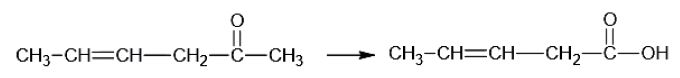1. a. I2 and NaOH solution
2. b. Tollen’s reagent
3. c. Sn and NaOH solution
4. d. Benzoyl peroxide

Solution: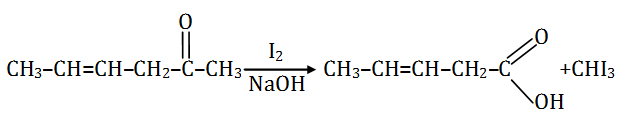I2/NaOH, since the byproduct formed is in a gaseous state and hence can be easily separated.

Question 37:. Which of the following is least soluble in water at 298 K?

1. a. (CH3)3N
2. b. CH3NH2
3. c. C6H5NH2
4. d. (CH3)2NH

Solution:

(CH3)3N shows no H-bonding hence it is least soluble.

Question 38:. If aniline is treated with 1:1 mixture of conc. HNO3 and con. H2SO4, p-nitroaniline and m-nitroaniline are formed nearly in equal amounts. This is due to

1. a. Protonation of -NH2 which causes deactivation of the benzene ring
2. b. m-directing property of -NH2 group
3. c. Isomerisation of some p-nitroaniline into m-nitroaniline
4. d. m & p directing property of -NH2 group

Solution:

In presence of an acid, aniline gets protonated to anilinium ion which is meta directing.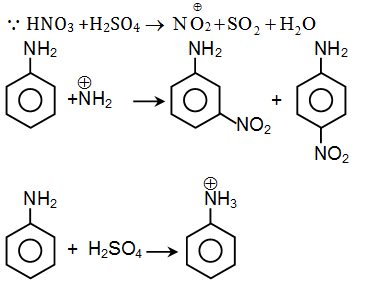Question 39:. In nucleic acids, the nucleotides are joined together by

Solution:

Nucleic acids and nucleotides are joined by a phosphodiester linkage.

A nucleotide has three components nitrogenous base, a pentose sugar; ribose in case of RNA and deoxyribose in case of DNA. A nitrogenous base is linked to the pentose sugar through an N-glycosidic linkage to form a nucleoside. The nucleotides are linked through 3-5 phosphodiester linkage to form dinucleotide.

Question 40:. Which of the following is generally water-insoluble?

1. a. Vitamin-C
2. b. Fibrous protein
3. c. Glycine
4. d. Amylose

Solution:

Vitamin A, D, E, K are water-insoluble vitamins. While B, C are water-soluble vitamins.

Question 41:. Relative lowering of the vapour pressure of a dilute solution of glucose dissolved in 1kg of water is 0.002. The molality of the solution is

1. a. 0.222
2. b. 0.004
3. c. 0.021
4. d. 0.111

Solution:

(P0-P)/P0 = (w2/m2)×(m1/w1)

0.002 = (w2/m2)×(18/1000)

w2/m2 = 0.11 mole

Molality = (w2/m2)×(1000/w1)

= 0.11×1000/1000

= 0.11m

Question 42:. One-litre solution of MgCl2 is electrolyzed completely by passing a current of 1A for 16 min 5 sec. The original concentration of MgCl2 solution was (Atomic mass of Mg = 24)

1. a. 5 × 10–2 M
2. b. 5 × 10–3 M
3. c. 1.0 × 10–2 M
4. d. 0.5 × 10–3 M

Solution:

m = EIZ/96500

= 12×1×965/96500

= 12×10-2g

Molarity = M = (w2/m2)×(1000/vm1)

= (12×10-2/24)×(1000/1000)

= 0.5×10-2

= 5×10-3m

Question 43:. An aqueous solution of CuSO4 is subjected to electrolysis using inert electrodes. The pH of the solution will

1. a. Remain unchanged
2. b. increase
3. c. increase or decrease depending on the strength of the current
4. d. decrease

Solution:

We can find the answer by writing equations of electrolysis of CuSO4.

At cathode: Cu2+(aq) +2e- Cu(s)

At anode: 2H2O O2+4H++4e-

Production of H+ ions will decrease PH.

Question 44:. Given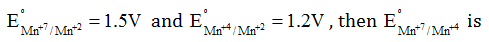1. a. 0.1 V
2. b. 0.3 V
3. c. 2.1 V
4. d. 1.7 V

Solution:

Mn+7+3e- Mn4+

G0 = -nFE0

G0 = -5F 1.5

Mn+4+2e- Mn+2

G0 = -2F 1.2

Required equation

Mn+4+3e- Mn4+

-3FE0 = -7.5F+2.4F

E0 = 1.7V

Question 45:. The plot of t1/2 v/s [R]0 for a reaction is a straight-line parallel to the x-axis. The unit for the rate constant of this reaction is

1. a. mol L–1 s–1
2. b. mol L–1 s
3. c. s–1
4. d. L mol–1 s–1

Solution:

t1/2 is independent of the initial concentration of the reactant and is first-order reaction.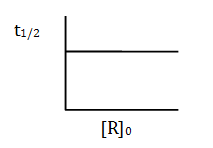Question 46:. The metal nitrate that liberates NO2 on heating

1. a. LiNO3
2. b. NaNO3
3. c. RbNO3
4. d. KNO3

Solution:

4LiNO3 2Li2O+4NO2+O2

LiNO3 is a metal nitrate that librates NO2 on heating.

Question 47:. Which of the following is NOT true regarding the use of hydrogen as a fuel?

1. a. The combustible energy of hydrogen can be directly converted to electrical energy in a fuel cell.
2. b. High calorific value
3. c. Hydrogen gas can be easily liquefied and stored.
4. d. Combustion product is eco friendly.

Solution:

The critical temperature of hydrogen is low hence it cannot be easily liquefied. Its combustion is eco friendly, it does not liberate harmful gases on combustion. It has a high calorific value.

Question 48:. Resonance effect is not observed in

1. a. CH2=CH≡CN
2. b. CH2=CH-CH=CH2
3. c. CH2=CH-CH2-NH2
4. d. CH2=CH-Cl

Solution:

For lone pair nitrogen atom, no conjugations of Pi electrons are present hence will not show resonance.

Question 49:. 2-butyne is reduced to trans-but-2-ene using

1. a. Na in liq. NH3
2. b. H2 Ni
3. c. Zn in dil. HCl
4. d. H2 Pd–C

Solution:

2-butyne can be reduced to transbut-2-ene using Na in liq NH3.

This reaction is called Birch reduction.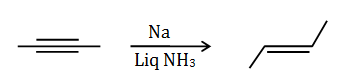Question 50:. Eutrophication causes

1. a. Reduction in water pollution
2. b. Increase of nutrients in the water
3. c. Decreases BOD
4. d. Reduction in dissolved oxygen

Solution:

Eutrophication refers to an excessive increase in minerals and nutrients in an aquatic system, resulting in excess of algae growth and reduction in dissolved oxygen.

Question 51:. Addition of excess AgNO3 to an aqueous solution of 1 mole of PdCl2+4NH3, gives 2 moles of AgCl. The conductivity of this solution corresponds to

1. a. 1 : 3 electrolyte
2. b. 1 : 1 electrolyte
3. c. 1 : 4 electrolyte
4. d. 1 : 2 electrolyte

Solution:

[Pd(CH3)4]Cl2 [Pd(NH3)4]2++2Cl-

It is 1:2 i.e. AB2 type of electrolyte.

Question 52:. The formula of Penta aquanitrato chromium (III) nitrate is:

1. a. [Cr(H2O)6](NO2)2
2. b. [Cr(H2O)6](NO3)3
3. c. [Cr(H2O)5NO2]NO3
4. d. [Cr(H2O)5NO3](NO3)2

Solution:

The formula of penta aquanitrato chromium III nitrate is [Cr(H2O)5(NO3)](NO3)2.

The prefix penta indicates the presence of 5 H2O ligands. The names of ligands are written in alphabetical order.

Question 53:. Which of the following halide undergoes hydrolysis on warming with water/aqueous NaOH?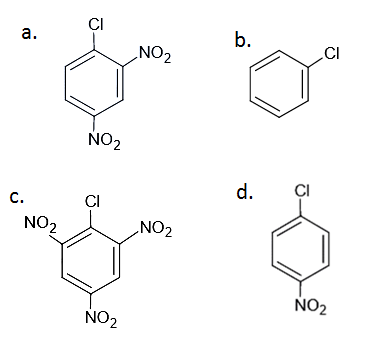Solution:

As the number of EWG at ortho and para position increases, reactivity towards nucleophilic substitution reaction increases.

Question 54:. The compound having the longest C-Cl bond is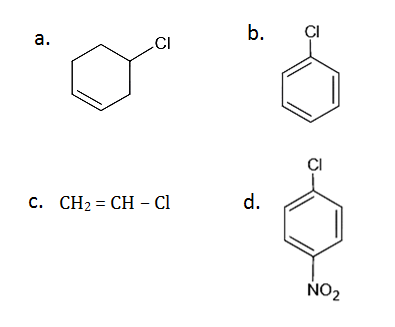Solution:

In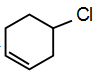this compound the lone pairs of chlorine atom are not in resonance while in remaining structures, lone pairs are in resonance. Hence, C-Cl bond has double bond characters. While in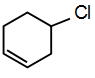this structure bond is purely sp3 hybridized.

Question 55:. The alkyl halides required to prepare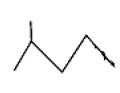by Wurtz reaction are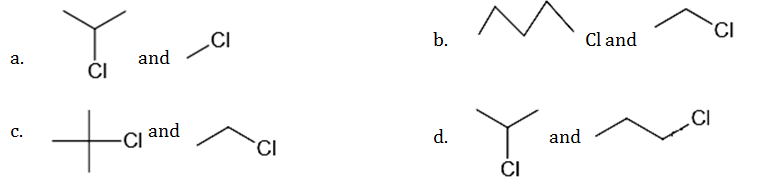Solution: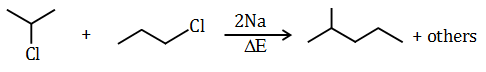Question 56:. Which is a wrong statement?

1. a. e-Ea/RT gives the fraction of reactant molecules that are activated at the given temp
2. b. Rate constant k = Arrhenius constant A ; if Ea = 0.
3. c. Presence of catalyst will not alter the value of Ea.
4. d. In k vs 1/T plot is a straight line.

Solution:

ln k v/s 1/T graph is a straight line graph but the presence of catalyst will alter the value of Ea.

Question 57:. 1L of 2M CH3COOH is mixed with 1L of 3M C2H5OH to form an ester. The rate of the reaction with respect to the initial rate when each solution is diluted with an equal volume of water will be

1. a. 2 times
2. b. 0.25 times
3. c. 4 times
4. d. 0.5 times

Solution:

For finding rate of reaction we have to write the equation of esterification.

CH3COOH+C2H5OH CH3COOC2H5+H2O

r = k [CH3COOH][C2H5OH]

Order = 2

When equal volumes of two solutions are mixed, the concentration of the solutions reduces to half of the initial value. Hence, the rate of reaction gets reduced to 1/4 of the initial rate.

Question 58:. Which of the following is an example of homogeneous catalysis?

1. a. Oxidation of SO2 in the contact process
2. b. Oxidation of NH3 in Oswald’s process
3. c. Manufacture of NH3 by Haber’s process
4. d. Oxidation of SO2 in the lead chamber process

Solution:

When both reactants and catalysts are in the same phase, then the catalysis is homogeneous.

2SO2(g) +O2(g) 2SO3 (g)

Question 59:. Critical Micelle concentration for a soap solution is 1.5×10-4 mol L-1. Micelle formation is possible only when the concentration of soap solution in mol L-1 is:

1. a. 4.6×10-5
2. b. 2.0×10-3
3. c. 1.1×10-4
4. d. 7.5×10-5

Solution:

Micelles are associated with colloids when the temperature increases above kraft temperature then particles get associated with each other and get into a colloidal range called ‘micelle’. For the formation of micelles, the concentration of soap should exceed CMC value.

Question 60: Oxidation state of copper is +1 in

1. a. Cuprite
2. b. Malachite
3. c. Chalcopyrite
4. d. Azurite

Solution:

In Cu2O cuprite oxidation state of Cu is +1.

Cu2O let oxidation state of Cu is x

2x+(-2) = 0

2x = 2

x = 2/2

x = +1

### KCET 2019 Chemistry Question Paper with Solutions